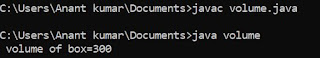## Tuesday, 17 July 2018

### Unions used in C programming language and uses union of structure

Union is the types of user define data structure which is same as like the structure but engaged style of both union and structure is totally different. As we knows that structure is used for storing different types of variable but each variable is stored in different spaces of memory whereas the union enables to store the different variable in same space of memory .the declaration of union is some similar to the structure used in C.The size of union is declared according to the size of variable of the union.

### Declaration of union

union  a
{
short int x ;
char  ;
};
union a student ;

### Program to uses union in C :

/* simple program to use union */
# include <stdio.h>
void main ()
{
union x
{
int i ;
char ch ;
};
union a student ;
student.i=100 ;
printf ("\n student.i=%d", student.i) ;
printf ("student.ch = %d\n",student.ch) ;
printf ("student.ch = %d\n",student.ch) ;
printf ("student.ch =%d\n",student.ch) ;
}

As you seen in the written simple program we have declare a variable union x , and then a variable student for the union x thus it looks similar to the declaration of a structure variable in C. If you write any program for storing information about student of schools then you have to input different information about the student then you uses structure or union but there is too much wastage of memory in case of structure so for this we need the union .

### Union of structure :

As we uses nested if else statement in basics of C here we uses structure nested into union and there are a union can be also nested into an another union.

/* structure nested in union */
# include <stdio.h>
void main ()
{
struct x
{
int i ;
char ch ;
};
struct y
{
int j ;
char ab ;
};
union z
{
struct x student ;
struct y values ;
};
union z odd ;
odd.student.i =102 ;
odd.values.ab = 0 ;
odd.values.ab = 55 ;
printf ('\n%d",odd.student.i) ;
printf ('\n%d",odd.values.j) ;
printf ('\n%d",odd.student.ch) ;
printf ('\n%d",odd.values.ab) ;
printf ('\n%d",odd.student.ch) ;
printf ('\n%d",odd.values.ab) ;
}

This is way by which we can declare a union variable in C . Read this before this structure in c.